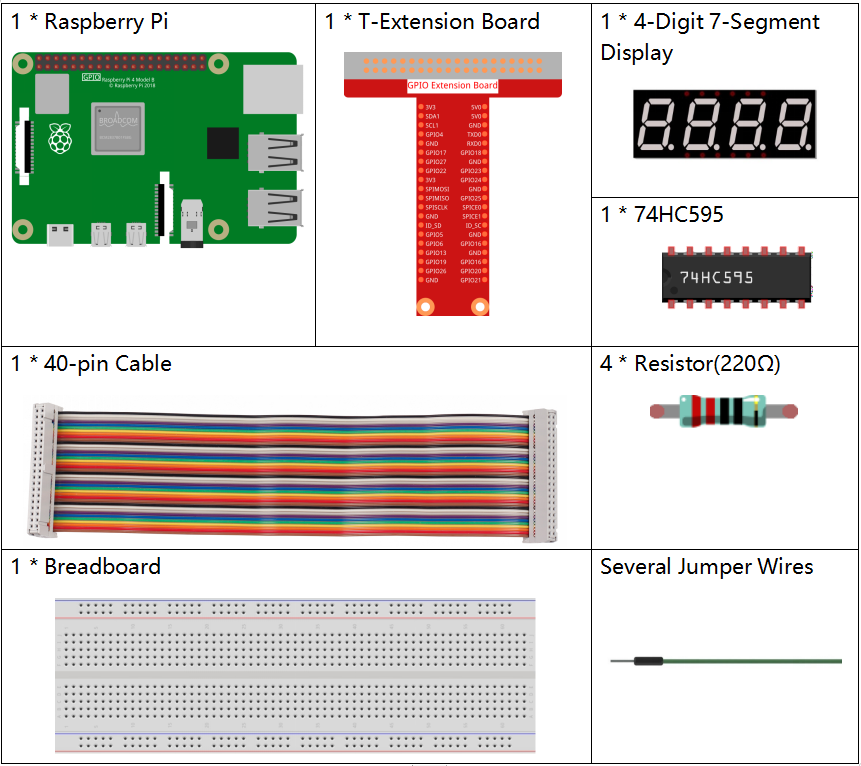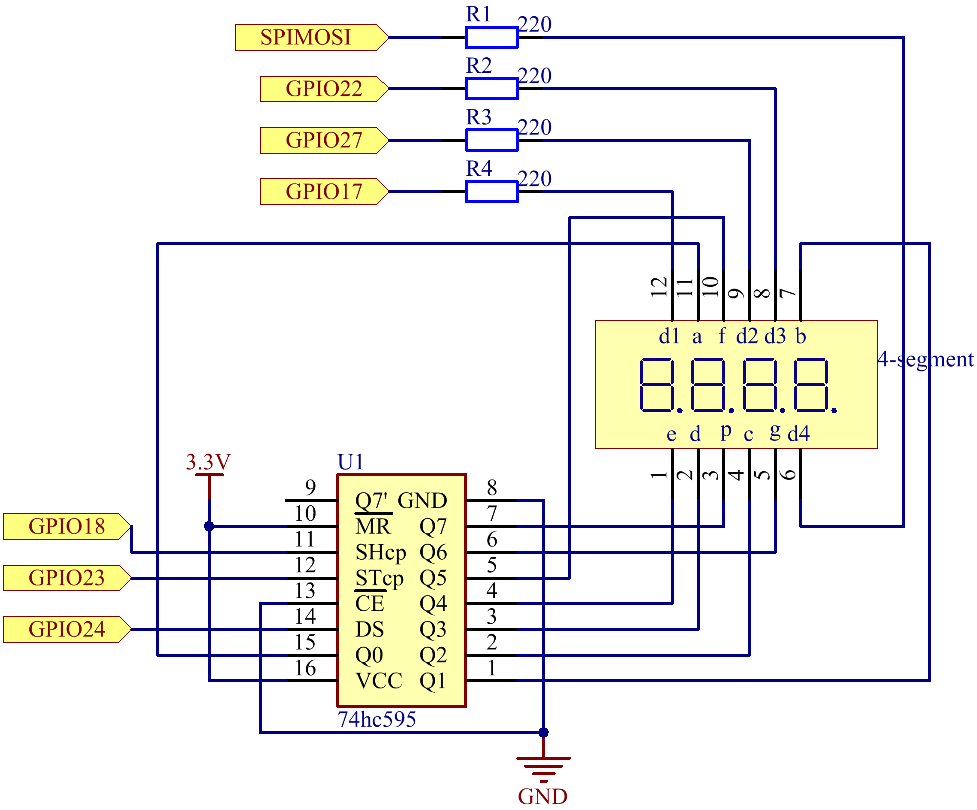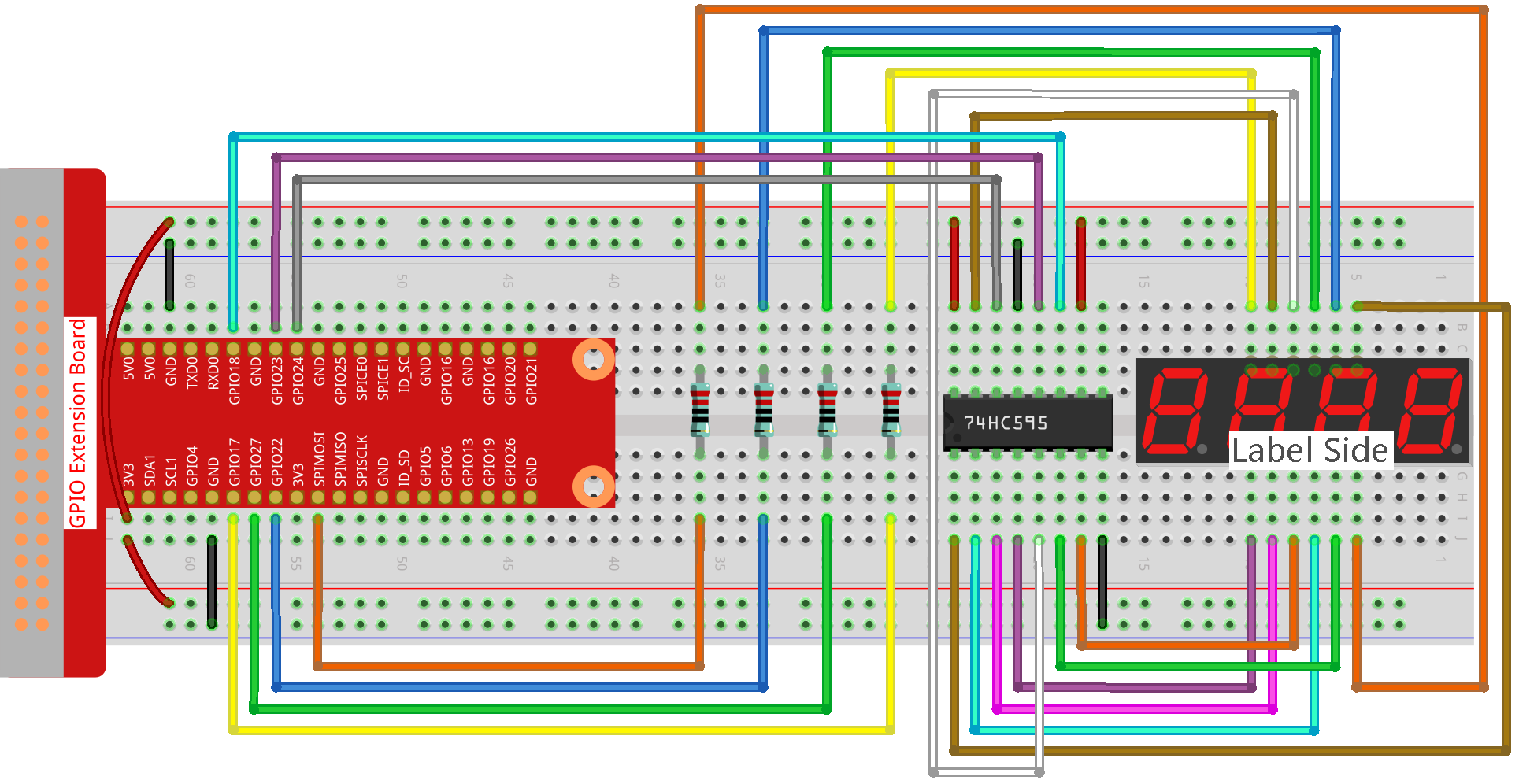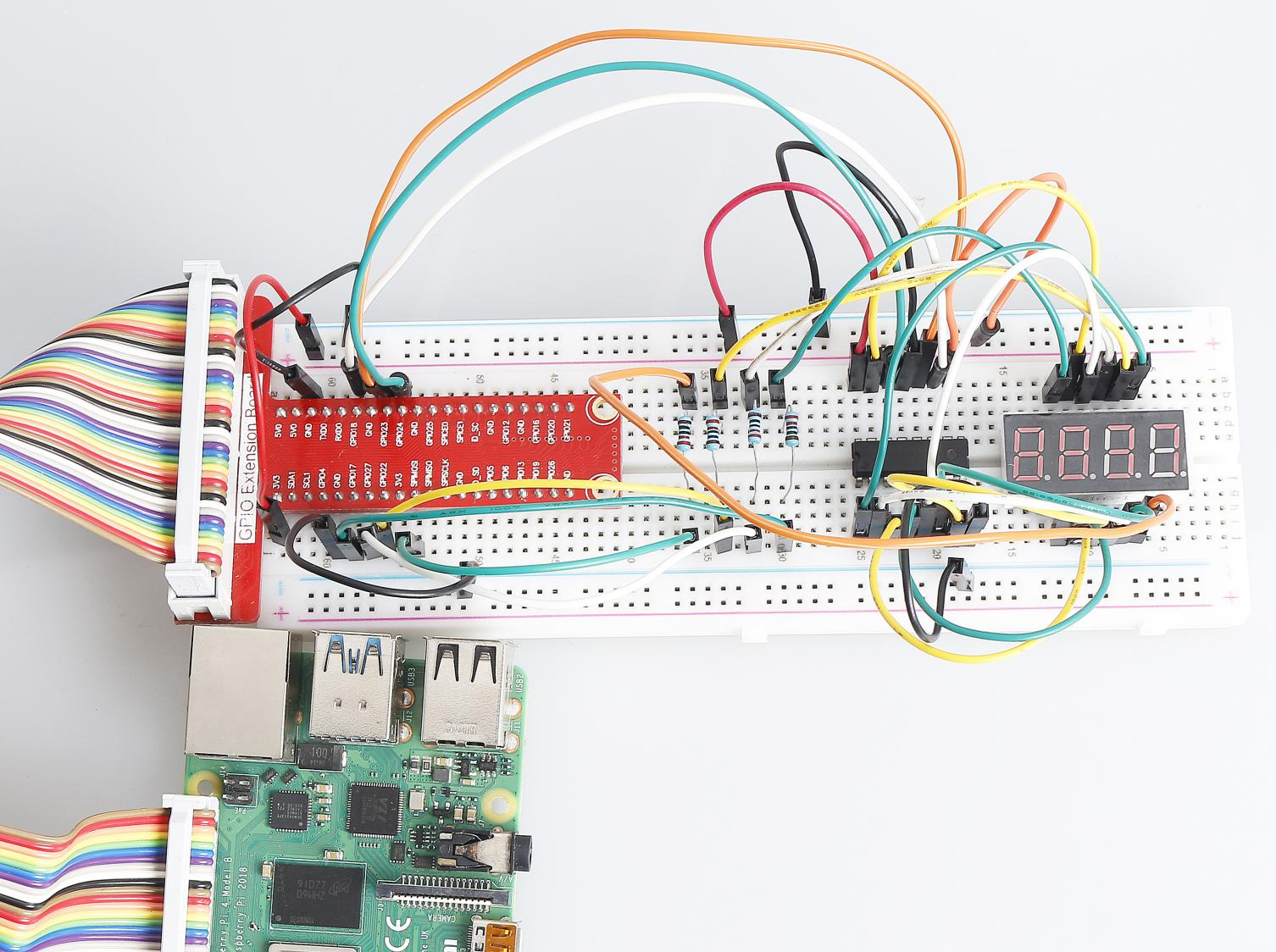# 1.1.5 4-Digit 7-Segment Display¶

## Introduction¶

Next, follow me to try to control the 4-digit 7-segment display.

## Required Components¶

In this project, we need the following components.Name

ITEMS IN THIS KIT

Raphael Kit

337

Raphael Kit

COMPONENT INTRODUCTION

GPIO Extension Board

Jumper Wires

Resistor

4-Digit 7-Segment Display

-

74HC595

## Schematic Diagram¶

 T-Board Name physical wiringPi BCM GPIO17 Pin 11 0 17 GPIO27 Pin 13 2 27 GPIO22 Pin 15 3 22 SPIMOSI Pin 19 12 10 GPIO18 Pin 12 1 18 GPIO23 Pin 16 4 23 GPIO24 Pin 18 5 24## Experimental Procedures¶

Step 1: Build the circuit.Step 2: Go to the folder of the code.

```cd ~/raphael-kit/python/
```

Step 3: Run the executable file.

```sudo python3 1.1.5_4-Digit.py
```

After the code runs, the program takes a count, increasing by 1 per second, and the 4 digit display displays the count.

Code

Note

You can Modify/Reset/Copy/Run/Stop the code below. But before that, you need to go to source code path like `raphael-kit/python`. After modifying the code, you can run it directly to see the effect.

```import RPi.GPIO as GPIO
import time

SDI = 24
RCLK = 23
SRCLK = 18

placePin = (10, 22, 27, 17)
number = (0xc0, 0xf9, 0xa4, 0xb0, 0x99, 0x92, 0x82, 0xf8, 0x80, 0x90)

counter = 0
timer1 = 0

def clearDisplay():
for i in range(8):
GPIO.output(SDI, 1)
GPIO.output(SRCLK, GPIO.HIGH)
GPIO.output(SRCLK, GPIO.LOW)
GPIO.output(RCLK, GPIO.HIGH)
GPIO.output(RCLK, GPIO.LOW)

def hc595_shift(data):
for i in range(8):
GPIO.output(SDI, 0x80 & (data << i))
GPIO.output(SRCLK, GPIO.HIGH)
GPIO.output(SRCLK, GPIO.LOW)
GPIO.output(RCLK, GPIO.HIGH)
GPIO.output(RCLK, GPIO.LOW)

def pickDigit(digit):
for i in placePin:
GPIO.output(i,GPIO.LOW)
GPIO.output(placePin[digit], GPIO.HIGH)

def timer():
global counter
global timer1
timer1.start()
counter += 1
print("%d" % counter)

def loop():
global counter
while True:
clearDisplay()
pickDigit(0)
hc595_shift(number[counter % 10])

clearDisplay()
pickDigit(1)
hc595_shift(number[counter % 100//10])

clearDisplay()
pickDigit(2)
hc595_shift(number[counter % 1000//100])

clearDisplay()
pickDigit(3)
hc595_shift(number[counter % 10000//1000])

def setup():
GPIO.setmode(GPIO.BCM)
GPIO.setup(SDI, GPIO.OUT)
GPIO.setup(RCLK, GPIO.OUT)
GPIO.setup(SRCLK, GPIO.OUT)
for i in placePin:
GPIO.setup(i, GPIO.OUT)
global timer1
timer1.start()

def destroy():   # When "Ctrl+C" is pressed, the function is executed.
global timer1
GPIO.cleanup()
timer1.cancel()  # cancel the timer

if __name__ == '__main__':
setup()
try:
loop()
except KeyboardInterrupt:
destroy()
```

Code Explanation

```placePin = (10, 22, 27, 17)
```

These four pins control the common anode pins of the four-digit 7-segment displays.

```number = (0xc0, 0xf9, 0xa4, 0xb0, 0x99, 0x92, 0x82, 0xf8, 0x80, 0x90)
```

A segment code array from 0 to 9 in hexadecimal (common anode).

```def clearDisplay():
for i in range(8):
GPIO.output(SDI, 1)
GPIO.output(SRCLK, GPIO.HIGH)
GPIO.output(SRCLK, GPIO.LOW)
GPIO.output(RCLK, GPIO.HIGH)
GPIO.output(RCLK, GPIO.LOW)
```

Write “1” for eight times in SDI., so that the eight LEDs on the 7-segment Dispaly will turn off so as to clear the displayed content.

```def pickDigit(digit):
for i in placePin:
GPIO.output(i,GPIO.LOW)
GPIO.output(placePin[digit], GPIO.HIGH)
```

Select the place of the value. there is only one place that should be enable each time. The enabled place will be written high.

```def loop():
global counter
while True:
clearDisplay()
pickDigit(0)
hc595_shift(number[counter % 10])

clearDisplay()
pickDigit(1)
hc595_shift(number[counter % 100//10])

clearDisplay()
pickDigit(2)
hc595_shift(number[counter % 1000//100])

clearDisplay()
pickDigit(3)
hc595_shift(number[counter % 10000//1000])
```

The function is used to set the number displayed on the 4-digit 7-segment Dispaly.

First, start the fourth segment display, write the single-digit number. Then start the third segment display, and type in the tens digit; after that, start the second and the first segment display respectively, and write the hundreds and thousands digits respectively. Because the refreshing speed is very fast, we see a complete four-digit display.

```timer1 = threading.Timer(1.0, timer)
timer1.start()
```

The module, threading is the common threading module in Python，and Timer is the subclass of it. The prototype of code is:

```class threading.Timer(interval, function, args=[], kwargs={})
```

After the interval, the function will be run. Here, the interval is 1.0，and the function is timer(). start () means the Timer will start at this point.

```def timer():
global counter
global timer1